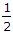# Civil Engineering - Applied Mechanics

### Exercise :: Applied Mechanics - Section 3

31.

The resultant of two forces acting at right angles is 34 kg and acting at 60° is 70 kg. The forces are

 A. 1 kg and 4 kg B. 2 kg and 3 kg C. 3 kg and 5 kg D. 3 kg and 5 kg E. 3 kg and 5 kg.

Explanation:

No answer description available for this question. Let us discuss.

32.

The velocity of a body fallen from height h, on reaching the ground is given by

 A. v = 2gh B. v = 2gh2 C. v = 2gh D. v = 1/2gh E. h2/2g

Explanation:

No answer description available for this question. Let us discuss.

33.

Two particles have been projected at angles 64° and 45° to the horizontal. If the velocity of projection of first is 10 m/sec, the velocity of projection of the other for equal horizontal ranges is

 A. 9.3 m/sec B. 8.3 m/sec C. 7.3 m/sec D. 6.3 m/sec.

Explanation:

No answer description available for this question. Let us discuss.

34.

μ is coefficient of friction. A wheeled vehicle travelling on a circular level track will slip and overturn simultaneously if the ratio of its wheel distance to the height of its centroid, is

 A. μ B. 2μ C. 3μ D.μ.

Explanation:

No answer description available for this question. Let us discuss.

35.

The necessary condition of equilibrium of a body, is :

 A. algebraic sum of horizontal components of all the forces must be zero B. algebraic sum of vertical components of all the forces must be zero C. algebraic sum of the moments of the forces about a point must be zero D. all (a), (b) and (c).